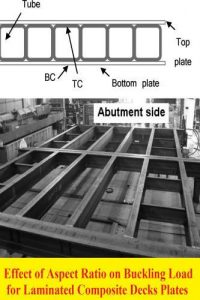اسم المؤلف
Dr. Osama Mohammed Elmardi Suleiman
التاريخ
3 ديسمبر 2020
المشاهدات
التقييم(لا توجد تقييمات)Loading...

بحث بعنوان
Effect of Aspect Ratio on Buckling Load for Laminated Composite Decks Plates
Dr. Osama Mohammed Elmardi Suleiman
Nile Valley University, Dept. of Mechanical Engineering, Sudan
e-mail: [email protected]
ABSTRACT: Finite element (FE) method is presented for the analysis of thin rectangular
laminated composite decks plates under the biaxial action of in – plane compressive loading.
The analysis uses the classical laminated plate theory (CLPT) which does not account for
shear deformations. In this theory it is assumed that the laminate is in a state of plane stress,
the individual lamina is linearly elastic, and there is perfect bonding between layers. The
classical laminated plate theory (CLPT), which is an extension of the classical plate theory
(CPT) assumes that normal to the mid – surface before deformation remains straight and
normal to the mid – surface after deformation. Therefore, this theory is only adequate for
buckling analysis of thin laminates. A Fortran program has been developed. New numerical
results are generated for in – plane compressive biaxial buckling which serve to quantify the
It is observed that the buckling load increases continuously with increasing aspect ratio but
the rate of increase is not uniform. This may be due to the effect of bending – extensional
twisting stiffness which increases the critical load. The buckling load is maximum for
clamped – clamped (CC), clamped – simply supported (CS) while minimum for simply –
simply supported (SS) boundary conditions. This means that as the plate becomes more
restrained, its resistance to buckling increases. The reason is that the structural stiffness
reduces due to its constrains.
KEYWORDS: Aspect ratio, biaxial buckling, classical laminated plate theory, finite element,
Fortran program, composite laminated decks plates
CONCLUDING REMARKS
A Fortran program based on finite elements (FE) has been developed for buckling
analysis of thin rectangular laminated decks plates using classical laminated plate theory
(CLPT). The problem of buckling loads of generally layered composite plates has been
studied. The problem is analyzed and solved using the energy approach, which is formulated
by a finite element model. In this method, quadrilateral elements are applied utilizing a four
noded model. Each element has three degrees of freedom at each node. The degrees of
freedom are: lateral displacement ( ), and rotation ( ) and ( ) about the and axes
respectively. The finite element model has been formulated to compute the buckling loads of
laminated plates with rectangular cross – section and to study the effects of lamination
scheme on the non – dimensional critical buckling loads of laminated composite plates. New
results have been presented. It is observed that the buckling load increases continuously with
increasing aspect ratio but the rate of increase is not uniform. This may be due to the effect of
bending – extensional twisting stiffness which increases the critical load.
كلمة سر فك الضغط : books-world.net